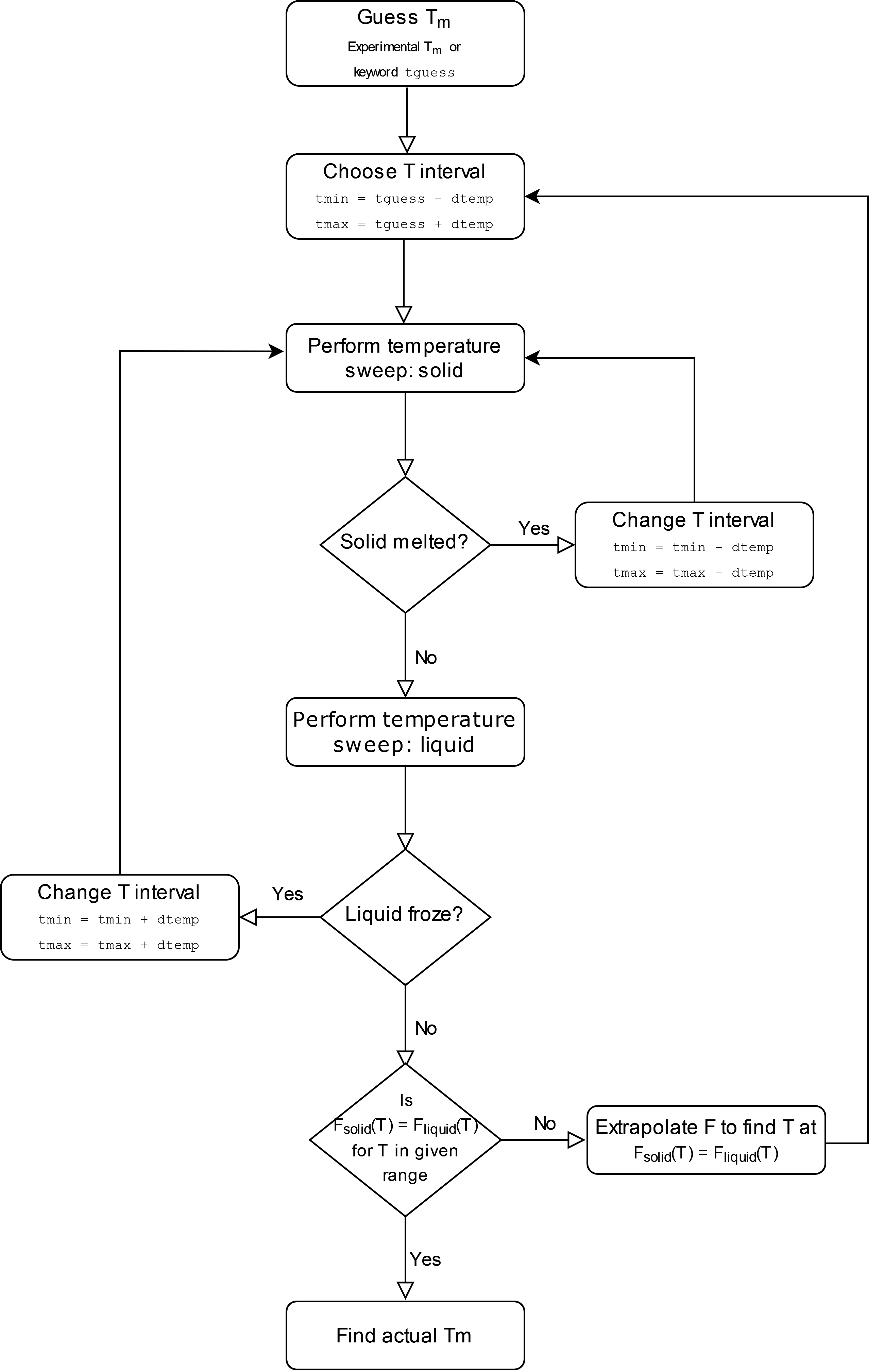# Example 04: Automated calculation of melting temperature#

In Example 03, we calculated the melting temperature for Cu. The same can be done in a fully automated way using Calphy.

The calculation block gives the input conditions at which the calculation is carried out. First of all, the mode is melting_temperature. This mode is special, and needs much less input information than the other common modes. For example, options such as lattice and temperature are (generally; see below for specific cases) not needed.

The input file in this case is shown below:

calculations:
- element: Cu
mass: 63.546
md:
timestep: 0.001
mode: melting_temperature
n_equilibration_steps: 10000
n_iterations: 1
n_switching_steps: 15000
pair_coeff: '* * ../potentials/Cu01.eam.alloy Cu'
pair_style: eam/alloy
queue:
commands:
- conda activate calphy
cores: 4
scheduler: local
repeat:
- 5
- 5
- 5


Once the input file is set up, the calculation can be run using:

calphy -i input.yaml


A log file called calphy.log is also be produced. The log file contains a lot of information about the calculation. Here the most important ones are discussed. The important log file contents are prefixed with STATE. On running grep STATE calphy.log, the following output is produced.

calphy.helpers INFO     STATE: Temperature range of 1156.600000-1556.600000 K
calphy.helpers INFO     STATE: Tm = 1340.39 K +/- 0.00 K, Exp. Tm = 1356.60 K


The calculated melting temperature for this interatomic potential is 1340 K.

## How does it work?#

A flowchart of the automated melting temperature calculation is shown below:## FAQs#

• How can I tune the initial guess temperature?
The initial guess temperature can be tuned using the keyword tguess in the md block.
• How can I tune the width of the temperature range?
The width of the temperature scan range can be tuned using the keyword dtemp in the md block.
• What if the system undergoes a solid-solid phase transition before melting?
The lattice that calphy automatically chooses for the solid is the ground state. For some elements, for example Ti, a solid-solid phase transition occurs before melting. HCP Ti transforms to BCC Ti and then to liquid. Therefore to use the automated method, the solid lattice has to be specified using the lattice keyword.
• How can I calculate melting temperature at non zero pressure?
The required pressure can be specified using the pressure keyword. Note that specifying tguess could help speed up the calculation.